# Array To Matrix VI

## LabVIEW 2018 Help

Edition Date: March 2018
Part Number: 371361R-01
View Product InfoLabVIEW 2016 HelpLabVIEW 2017 HelpLabVIEW 2018 HelpLabVIEW 2019 HelpLabVIEW 2020 Help

Owning Palette: Array Functions

Requires: Base Development System

Converts an array to a matrix of elements of the same type as the array elements. Wire data to the Real 2D Array input to determine the polymorphic instance to use or manually select the instance.

Use the pull-down menu to select an instance of this VI.

 Select an instance To Real MatrixTo Column VectorTo Complex MatrixTo Complex Column Vector

## To Real Matrix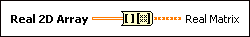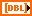Real 2D Array specifies a two-dimensional array.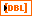The elements in Real Matrix are of the same type as the elements in Real 2D Array. The order of the elements in Real Matrix is the same as the Real 2D Array order of the elements.

## To Column Vector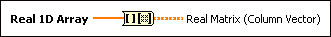Real 1D Array specifies a one-dimensional array.The elements in Real Matrix (Column Vector) are of the same type as the elements in Real 1D Array. The order of the elements in Real Matrix (Column Vector) is the same as the Real 1D Array order of the elements.

## To Complex Matrix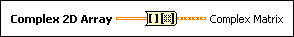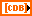Complex 2D Array specifies a complex two-dimensional array.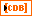The elements in Complex Matrix are of the same type as the elements in Complex 2D Array. The order of the elements in Complex Matrix is the same as the Complex 2D Array order of the elements.

## To Complex Column Vector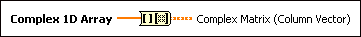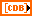Complex 1D Array specifies a complex one-dimensional array.The elements in Complex Matrix (Column Vector) are of the same type as the elements in Complex 1D Array. The order of the elements in Complex Matrix (Column Vector) is the same as the Complex 1D Array order of the elements.

## Array To Matrix Details

If the array elements are not real or complex double-precision floating-point numbers, LabVIEW converts the array elements to the closest data type.

LabVIEW stores the elements of 1D arrays in the first column of the matrix.

## Examples

Refer to the following VIs for examples of using the Array To Matrix VI:

• Matrix Fundamentals VI: labview\examples\Mathematics\Linear Algebra
• Matrix to a Power VI: labview\examples\Mathematics\Linear Algebra